# Design and Cosimulate Control of High-Fidelity Distillation Tower with Aspen Plus Dynamics

This example shows how to design a model predictive controller in MATLAB for a high-fidelity distillation tower model built in Aspen Plus Dynamics®. The controller performance is then verified through cosimulation between Simulink and Aspen Plus Dynamics.

### Distillation Tower

The distillation tower uses 29 ideal stages to separate a mixture of benzene, toluene, and xylenes (represented by p-xylene). The distillation process is continuous. The equipment includes a reboiler and a total condenser as shown below: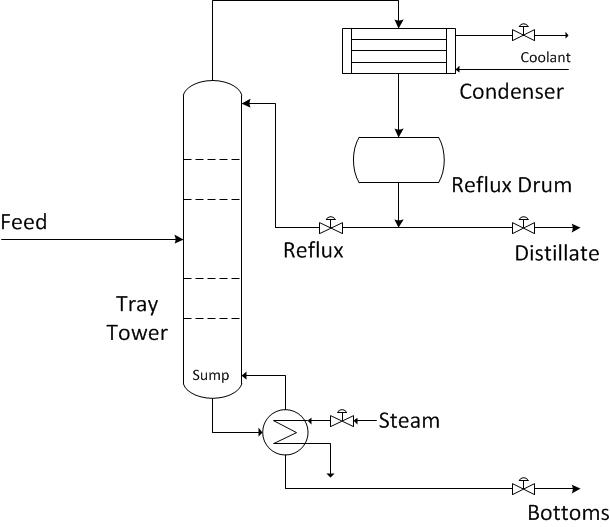The distillation tower operates at a nominal steady-state condition:

• The feed stream contains 30% of benzene, 40% of toluene and 30% of xylenes.

• The feed flow rate is 500 kmol/hour.

• To satisfy the distillate purity requirement, the distillate contains 95% of benzene.

• To satisfy the requirement of recovering 95% of benzene in the feed, the benzene impurity in the bottoms is 1.7%.

The control objectives are listed below, sorted by their importance:

1. Hold the tower pressure constant.

2. Maintain 5% of toluene in the distillate (it is equivalent to maintain 95% of benzene in the distillate because the distillate only contains benzene and toluene).

3. Maintain 1.7% of the benzene in the bottoms.

4. Keep liquid levels in the sump and the reflux drum within specified limits.

### Build High-Fidelity Plant Model in Aspen Plus Dynamics

Use an Aspen Plus `RADFRAC` block to define the tower's steady-state characteristics. In addition to the usual information needed for a steady-state simulation, you must specify tray hydraulics, tower sump geometry, and the reflux drum size. The trays are a sieve design spaced 18 inches apart. All trays have a 1.95 m in diameter with a 5 cm weir height. Nominal liquid depths are 0.67 m and 1.4875 m in the horizontal reflux drum and sump respectively.

The steady-state model is ported to Aspen Plus Dynamics (APD) for a flow-driven simulation. This neglects actuator dynamics and assumes accurate regulation of manipulated flow rates. By default, APD adds PI controllers to regulate the tower pressure and the two liquid levels. In this example, the default PI controllers are intentionally removed.

The APD model of the high-fidelity distillation tower is shown below: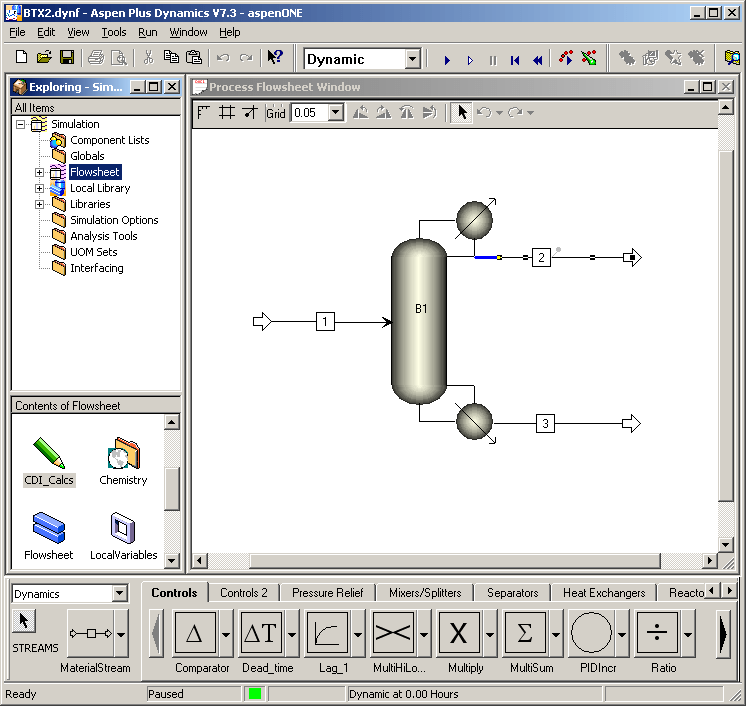Model Predictive Controller requires an LTI model of the plant. In this example, the plant inputs are:

1. Condenser duty (W)

2. Reboiler duty (W)

3. Reflux mass flow rate (kg/h)

4. Distillate mass flow rate (kg/h stream #2)

5. Bottoms mass flow rate (kg/h stream #3)

6. Feed molar flow rate (kmol/h stream #1)

The plant outputs are:

1. Tower pressure (in the condenser: stage 1, bar)

2. Reflux drum liquid level (m)

3. Sump liquid level (m)

4. Mass fraction toluene in the distillate

5. Mass fraction benzene in the bottoms

Aspen Plus Dynamics provides a Control Design Interface (CDI) tool that linearizes a dynamic model at a specified condition.

The following steps are taken to obtain the linear plant model in Aspen Plus Dynamics.

`Step 1`: Add a script to the APD model under the `Flowsheet` folder. In this example, the script name is `CDI_Calcs` (as shown above) and it contains the following APD commands:

```Set Doc = ActiveDocument set CDI = Doc.CDI CDI.Reset CDI.AddInputVariable "blocks(""B1"").condenser(1).QR" CDI.AddInputVariable "blocks(""B1"").QrebR" CDI.AddInputVariable "blocks(""B1"").Reflux.FmR" CDI.AddInputVariable "streams(""2"").FmR" CDI.AddInputVariable "streams(""3"").FmR" CDI.AddInputVariable "streams(""1"").FR" CDI.AddOutputVariable "blocks(""B1"").Stage(1).P" CDI.AddOutputVariable "blocks(""B1"").Stage(1).Level" CDI.AddOutputVariable "blocks(""B1"").SumpLevel" CDI.AddOutputVariable "streams(""2"").Zmn(""TOLUENE"")" CDI.AddOutputVariable "streams(""3"").Zmn(""BENZENE"")" CDI.Calculate ```

`Step 2`: Initialize the APD model to the nominal steady-state condition.

`Step 3`: Invoke the script, which generates the following text files:

• `cdi_A.dat`, `cdi_B.dat`, `cdi_C.dat` define the A, B, and C matrices of a standard continuous-time LTI state-space model. D matrix is zero. The A, B, C matrices are sparse matrices.

• `cdi_list.lis` lists the model variables and their nominal values.

• `cdi_G.dat` defines the input/output static gain matrix at the nominal condition. The gain matrix is also a sparse matrix.

In this example, `cdi_list.lis` includes the following information:

```A matrix computed, number of non-zero elements = 1408 B matrix computed, number of non-zero elements = 26 C matrix computed, number of non-zero elements = 20 G matrix computed, number of non-zero elements = 30 Number of state variables: 120 Number of input variables: 6 Number of output variables: 5 Input variables: 1 -3690034.247458334 BLOCKS("B1").Condenser(1).QR 2 3819023.193875 BLOCKS("B1").QRebR 3 22135.96620144 BLOCKS("B1").Reflux.FmR 4 11717.39655353 STREAMS("2").FmR 5 34352.86345834 STREAMS("3").FmR 6 500 STREAMS("1").FR Output variables: 1 1.100022977953499 BLOCKS("B1").Stage(1).P 2 0.6700005140605662 BLOCKS("B1").Stage(1).Level 3 1.4875 BLOCKS("B1").SumpLevel 4 0.05002582161855798 STREAMS("2").Zmn("TOLUENE") 5 0.01705308738356429 STREAMS("3").Zmn("BENZENE") ```

The nominal values of the state variables listed in the file are ignored because they are not needed in the MPC design.

### Create Scaled and Reduced LTI State-Space Model

`Step 1`: Convert the CDI-generated sparse-matrices to a state-space model.

Load state-space matrices from the CDI data files to MATLAB workspace and convert the sparse matrices to full matrices.

```load mpcdistillation_cdi_A.dat load mpcdistillation_cdi_B.dat load mpcdistillation_cdi_C.dat A = full(spconvert(mpcdistillation_cdi_A)); B = full(spconvert(mpcdistillation_cdi_B)); C = full(spconvert(mpcdistillation_cdi_C)); D = zeros(5,6);```

It is possible that an entire sparse matrix row or column is zero, in which case the above commands are insufficient. Use the following additional checks to make sure A, B, and C have the correct dimensions:

```[nxAr,nxAc] = size(A); [nxB,nu] = size(B); [ny,nxC] = size(C); nx = max([nxAr, nxAc, nxB, nxC]); if nx > nxC C = [C, zeros(ny,nx-nxC)]; end if nx > nxAc A = [A zeros(nxAr,nx-nxAc)]; end if nx > nxAr nxAc = size(A,2); A = [A; zeros(nx-nxAr, nxAc)]; end if nxB < nx B = [B; zeros(nx-nxB,nu)]; end```

`Step 2`: Scale the plant signals.

It is good practice, if not essential, to convert plant signals from engineering units to a uniform dimensionless scale (e.g., 0-1 or 0-100%). One alternative is to define scale factors as part of a Model Predictive Controller design. This can simplify controller tuning significantly. For example, see, Using Scale Factors to Facilitate Weight Tuning.

In the present example, however, we will use a model reduction procedure prior to controller design, and we therefore scale the plant model, using the scaled model in both model reduction and controller design. We define a span for each input and output, i.e., the difference between expected maximum and minimum values in engineering units. Also record the nominal and zero values in engineering units to facilitate subsequent conversions.

```U_span = [2*(-3690034), 2*3819023, 2*22136, 2*11717, 2*34353, 2*500]; U_nom = 0.5*U_span; U_zero = zeros(1,6); Y_nom = [1.1, 0.67, 1.4875, 0.050026, 0.017053]; Y_span = [0.4, 2*Y_nom(2:5)]; Y_zero = [0.9, 0, 0, 0, 0];```

Scale the B and C matrices such that all input/output variables are expressed as percentages.

```B = B.*(ones(nx,1)*U_span); C = C./(ones(nx,1)*Y_span)';```

`Step 3`: Define the state-space plant model.

```G = ss(A,B,C,D); G.TimeUnit = 'hours'; G.u = {'Qc','Qr','R','D','B','F'}; G.y = {'P','RLev','Slev','xD','xB'};```

`Step 4`: Reduce model order.

Model reduction speeds up the calculations with negligible effect on prediction accuracy. Use the `hsvd` command to determine which states can be safely discarded. Use the `balred` function to remove these states and reduce model order.

```[hsv, baldata] = hsvd(G); order = find(hsv>0.01,1,'last'); Options = balredOptions('StateElimMethod','Truncate'); G = balred(G,order,baldata,Options);```

The original model has 120 states and the reduced model has only 16 states. Note that the `Truncate` option is used in the `balred` function to preserve a zero D matrix. The model has two poles at zero, which correspond to the two liquid levels.

### Test Accuracy of the Linear Plant Model

Before continuing with the MPC design, it is good practice to verify that the scaled LTI model is accurate for small changes in the plant inputs. To do so, you need to compare the response of the nonlinear plant in APD and the response of linear model `G`.

`Step 1`: To obtain the response of the nonlinear plant, create a Simulink model and add the Aspen Modeler Block to it.

The block is provided by Aspen Plus Dynamics in their `AMSimulink` library.

`Step 2`: Double-click the block and provide the location of the APD model.

The APD model information is then imported into Simulink. For large APD models, the importing process may take some time.

`Step 3`: Specify input and output signals in the AMSimulation block.

Use the same variable names and sequence as in the CDI script.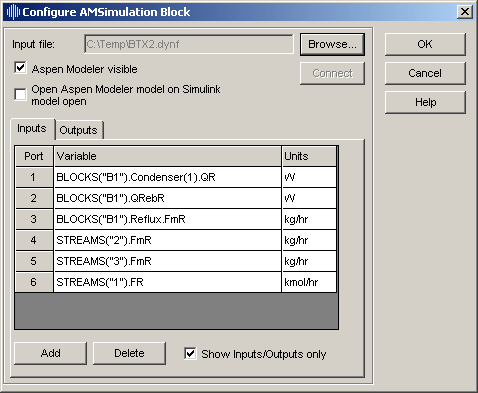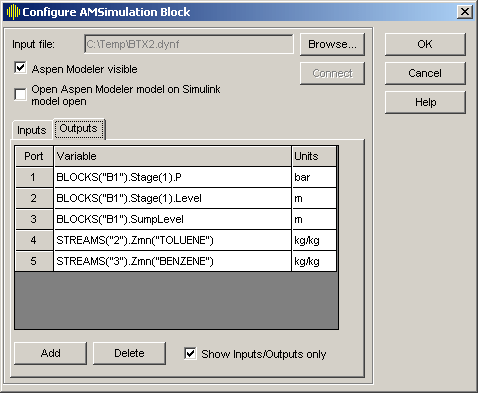The block now shows inports and outports for each signal that you defined.

`Step 4`: Expand the Simulink model with an input signal coming from the variable `Umat` and an output signal saved to variable `Ypct_NL`. Both variables are created in Step 5.

Since `Umat` is in the percentage units, the `Pct2Engr` block is implemented to convert from percentage units to engineering units.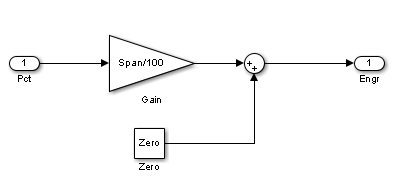Since `Ypct_NL` is in the percentage units, the "Engr2Pct" block is implemented to convert from engineering units to percentage units.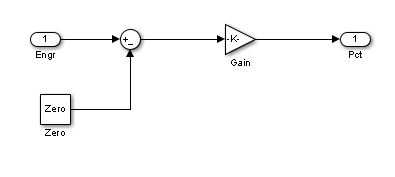With everything connected and configured, the model appears as follows: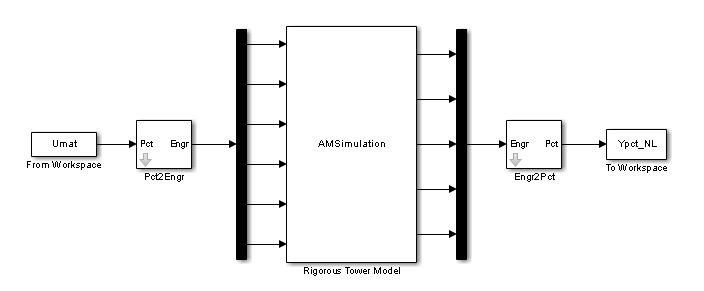`Step 5`: Verify linear model with cosimulation.

In this example, 1 percent increase in the scaled reflux rate (input #3) is used as the excitation signal to the plant.

```U_nom_pct = (U_nom - U_zero)*100./U_span; % Convert nominal condition from engineering units to percentages Y_nom_pct = (Y_nom - Y_zero)*100./Y_span; Tend = 1; % Simulation duration (1 hour) t = (0:1/60:Tend)'; % Sample period is 1 minute nT = length(t); Upct = ones(nT,1)*U_nom_pct; DUpct = zeros(nT,6); DUpct(:,3) = ones(nT,1); % Input signal where step occurs in channel #3```

The response of the linear plant model is computed using the `lsim` command and stored in variable `Ypct_L`.

```Ypct_L = lsim(G,DUpct,t); Ypct_L = Ypct_L + ones(nT,1)*Y_nom_pct;```

The response of the nonlinear plant is obtained through cosimulation between Simulink and Aspen Plus Dynamics. The excitation signal `Umat` is constructed as below. The result is stored in variable `Ypct_NL`.

`Umat = [t, Upct+DUpct];`

Compare the linear and nonlinear model responses.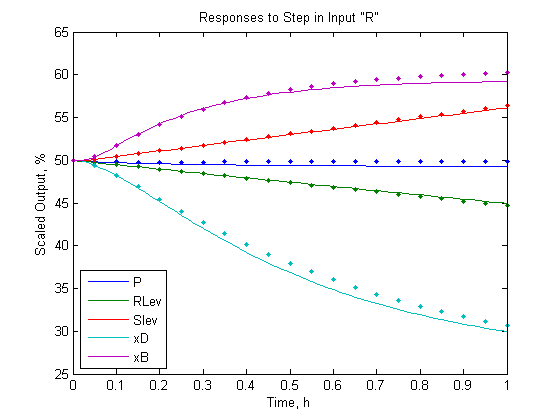The LTI model predictions track the nonlinear responses well. The amount of prediction error is acceptable. In any case, a Model Predictive Controller must be tuned to accommodate prediction errors, which are inevitable in applications.

You can repeat the above steps to verify similar agreement for the other five inputs.

### Design Model Predictive Controller

Given an LTI prediction model, you are ready to design a Model Predictive Controller. In this example, the manipulated variables are the first five plant inputs. The sixth plant input (feed flow rate) is a measured disturbance for feed-forward compensation. All the plant outputs are measured.

`Step 1`: Augment the plant to model unmeasured load disturbances.

Lacking any more specific details regarding load disturbances, it is common practice to assume an unmeasured load disturbance occurring at each of the five inputs. This allows the MPC state estimator to eliminate offset in each controlled output when a load disturbance occurs.

In this example, 5 unmeasured load disturbances are added to the plant model `G`. In total, there are now 11 inputs to the prediction model `Gmpc`: 5 manipulated variables, 1 measured disturbance, and 5 unmeasured disturbances.

```Gmpc = ss(G.A,G.B(:,[1:6,1:5]),G.C,zeros(5,11),'TimeUnit','hours'); InputName = cell(1,11); for i = 1:5 InputName{i} = G.InputName{i}; InputName{i+6} = [G.InputName{i}, '-UD']; end InputName{6} = G.InputName{6}; Gmpc.InputName = InputName; Gmpc.InputGroup = struct('MV',1:5,'MD',6,'UD',7:11); Gmpc.OutputName = G.OutputName;```

`Step 2`: Create an initial model predictive controller and specify sample time and horizons.

In this example, the controller sample period is 30 seconds. The prediction horizon is 60 intervals (30 minutes), which is large enough to make the controller performance insensitive to further increases of the prediction horizon. The control horizon is 4 intervals (2 minutes), which is relatively small to reduce computational effort.

```Ts = 30/3600; % sample time PH = 60; % prediction horizon CH = 4; % control horizon MPCobj = mpc(Gmpc,Ts,PH,CH); % MPC object```
```-->The "Weights.ManipulatedVariables" property of "mpc" object is empty. Assuming default 0.00000. -->The "Weights.ManipulatedVariablesRate" property of "mpc" object is empty. Assuming default 0.10000. -->The "Weights.OutputVariables" property of "mpc" object is empty. Assuming default 1.00000. ```

`Step 3`: Specify weights for manipulated variables and controlled outputs.

Weights are key tuning adjustments in MPC design and they should be chosen based on your control objectives.

There is no reason to hold a particular MV at a setpoint, so set the `Weights.ManipulatedVariables` property to zero:

`MPCobj.Weights.ManipulatedVariables = [0, 0, 0, 0, 0];`

The distillate product (MV #4) goes to storage. The only MV affecting downstream unit operations is the bottoms rate (MV #5). To discourage rapid changes in bottoms rate, retain the default weight of 0.1 for its rate of change. Reduce the other rate of change weights by a factor of 10:

`MPCobj.Weights.ManipulatedVariablesRate = [0.01, 0.01, 0.01, 0.01, 0.1];`

The control objectives provide guidelines to choose weights on controlled outputs:

1. The tower pressure must be regulated tightly for safety reasons and for minimizing upsets in tray temperatures and hydraulics. (objective #1)

2. The distillate composition must also be regulated tightly. (objective #2)

3. The bottoms composition can be regulated less tightly. (objective #3)

4. The liquid levels are even less important. (objective #4)

With these priorities in mind, weights on controlled outputs are chosen as follows::

`MPCobj.Weights.OutputVariables = [10, 0.1, 0.1, 1, 0.5];`

Scaling the model simplifies the choice of the optimization weights. Otherwise, in addition to the relative priority of each variable, you would also have to consider the relative magnitudes of the variables and choose weights accordingly.

`Step 4`: Specify nominal plant input/output values.

In this example, the nominal values are scaled as percentages. MPC controller demands that the nominal values for unmeasured disturbances must be zero.

```MPCobj.Model.Nominal.U = [U_nom_pct'; zeros(5,1)]; MPCobj.Model.Nominal.Y = Y_nom_pct';```

`Step 5`: Adjust state estimator gain.

Adjusting the state estimator gain affects the disturbance rejection performance. Increasing the state estimator gain (e.g. by increasing the gain of the input/output disturbance model) makes the controller respond more aggressively towards output changes (because the controller assumes the main source of the output changes is a disturbance, instead of measurement noise). On the other hand, decreasing the state estimator gain makes the closed-loop system more robust.

First, check whether using the default state estimator provides a decent disturbance rejection performance.

Simulate the closed-loop response to a 1% unit step in reflux (MV #3) in MATLAB. The simulation uses `G` as the plant, which implies no model mismatch.

```T = 30; % Simulation time r = Y_nom_pct; % Nominal setpoints v = U_nom_pct(6); % No measured disturbance SimOptions = mpcsimopt(MPCobj); SimOptions.InputNoise = [0 0 1 0 0]; % 1% unit step in reflux [y_L,t_L,u_L] = sim(MPCobj, T, r, v, SimOptions); % Closed-loop simulation```
```-->Converting model to discrete time. -->The "Model.Disturbance" property of "mpc" object is empty: Assuming unmeasured input disturbance #7 is integrated white noise. Assuming unmeasured input disturbance #8 is integrated white noise. Assuming unmeasured input disturbance #9 is integrated white noise. Assuming unmeasured input disturbance #10 is integrated white noise. Assuming unmeasured input disturbance #11 is integrated white noise. Assuming no disturbance added to measured output channel #1. Assuming no disturbance added to measured output channel #4. Assuming no disturbance added to measured output channel #5. Assuming no disturbance added to measured output channel #2. Assuming no disturbance added to measured output channel #3. -->The "Model.Noise" property of the "mpc" object is empty. Assuming white noise on each measured output channel. ```
```% plot responses f1 = figure(); subplot(2,1,1); plot(t_L,y_L,[0 t_L(end)],[50 50],'k--') title('Controlled Outputs, %') legend(Gmpc.OutputName,'Location','NorthEastOutside') subplot(2,1,2); plot(t_L,u_L(:,1:5),[0 t_L(end)],[50 50],'k--') title('Manipulated Variables, %') legend(Gmpc.InputName(1:5),'Location','NorthEastOutside') xlabel('Time, h')```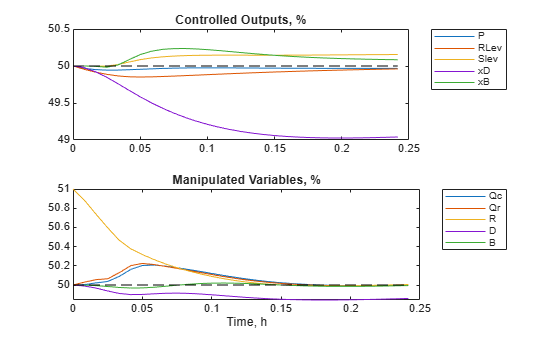The default estimator provides sluggish load rejection. In particular, the critical `xD` output drops to 49% and has just begun to return to the setpoint after 0.25 hours.

Secondly, increase the estimator gain by multiplying the default input disturbance model gain by a factor of 25.

```EstGain = 25; % factor of 25 Gd = getindist(MPCobj); % get default input disturbance model Gd_new = EstGain*Gd; % create new input disturbance model setindist(MPCobj,'Model',Gd_new); % set input disturbance model [y_L,t_L,u_L] = sim(MPCobj,T,r,v,SimOptions); % Closed-loop simulation```
```-->Converting model to discrete time. Assuming no disturbance added to measured output channel #1. Assuming no disturbance added to measured output channel #4. Assuming no disturbance added to measured output channel #5. Assuming no disturbance added to measured output channel #2. Assuming no disturbance added to measured output channel #3. -->The "Model.Noise" property of the "mpc" object is empty. Assuming white noise on each measured output channel. ```
```% plot responses f2 = figure(); subplot(2,1,1); plot(t_L,y_L,[0 t_L(end)],[50 50],'k--') title('Controlled Outputs, %') legend(Gmpc.OutputName,'Location','NorthEastOutside') subplot(2,1,2) plot(t_L,u_L(:,1:5),[0 t_L(end)],[50 50],'k--') title('Manipulated Variables, %') legend(Gmpc.InputName(1:5),'Location','NorthEastOutside') xlabel('Time, h')```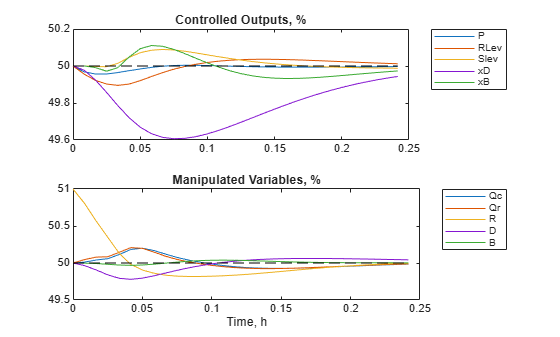Now, the peak deviation in `xD` is 50% less than the default case and `xD` returns to its setpoint much faster. Other variables also respond more rapidly.

Thirdly, look at the reflux response (#3 in the "Manipulated Variables" plot). Because the disturbance is a 1% unit step, the response begins at 51% and its final value is 50% at steady state. The reflux response overshoots by 20% (reaching 49.8%) before settling. This amount of overshoot is acceptable.

If the estimator gain were increased further (e.g. by a factor of 50), the controller overshoot would increase too. However, such aggressive behavior is unlikely to be robust when applied to the nonlinear plant model.

You can introduce other load disturbances to verify that disturbance rejection is now rapid in all cases.

Scaling the model also simplifies disturbance model tuning. Otherwise, you would need to adjust the gain of each channel in the disturbance model to achieve good disturbance rejection for all loads.

Generally, you next check the response to setpoint changes. If the response is too aggressive, you can use setpoint filter to smooth it. Setpoint filter has no effect on load disturbance rejection and thus can be tuned independently.

### Cosimulate MPC Controller and Nonlinear Plant

Use cosimulation to determine whether the MPC design is robust enough to control the nonlinear plant model.

`Step 1`: Add constraints to the MPC controller

Because the nonlinear plant model has input and output constraints during operation, MV and OV constraints are defined in the MPC controller as follows:

```MV = MPCobj.MV; OV = MPCobj.OV; % Physical bounds on MVs at 0 and 100 for i = 1:5 MV(i).Min = 0; MV(i).Max = 100; end MPCobj.MV = MV; % Keep liquid levels greater than 25% and less than 75% of capacity. for i = 2:3 OV(i).Min = 25; OV(i).Max = 75; end MPCobj.OV = OV;```

`Step 2`: Build Simulink model for cosimulation.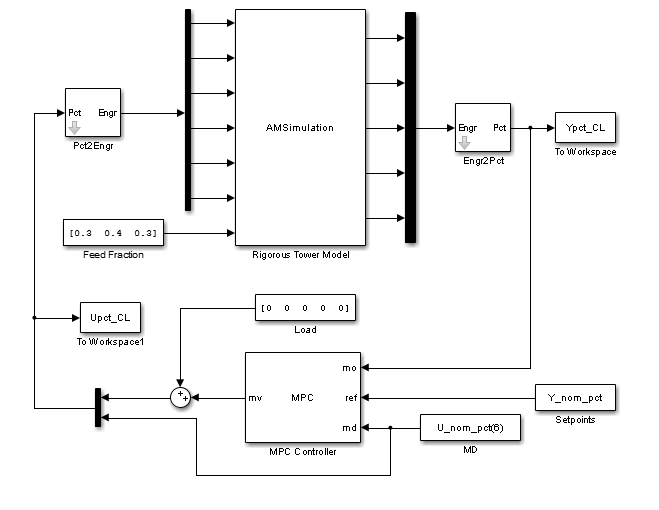The model can simulate 1% unit step in reflux (MV #3). It can also simulate a change in feed composition, which is a common disturbance and differs from the load disturbances considered explicitly in the design.

`Step 3`: Simulate 1% unit step in reflux (MV #3). Compare the closed-loop responses between using the linear plant model and using the nonlinear plant model.

Plot distillate product composition (`xD`) and the reflux rate (`R`):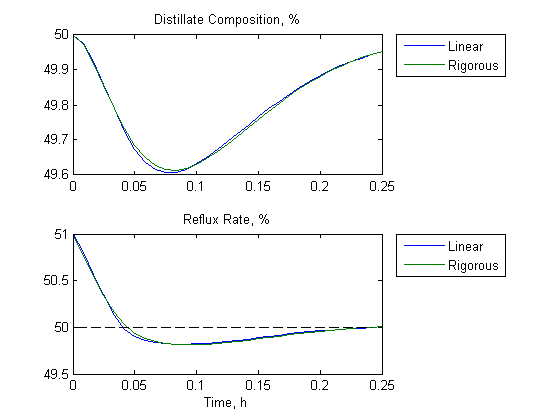In cosimulation, the model predictive controller rejects the small load disturbance in a manner almost identical to the linear simulation.

`Step 4`: Simulate a large decrease of benzene fraction (from 0.3 to 0.22) in the feed stream. Compare the closed-loop responses between using the linear and nonlinear plant models.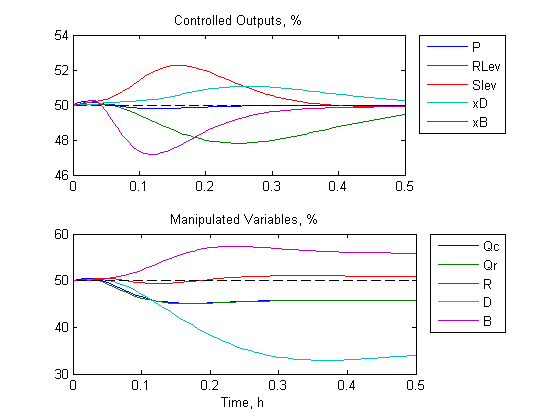The drop in benzene fraction requires a sustained decrease in the distillate rate and a corresponding increase in the bottoms rate. There are also sustained drops in the heat duties and a minor increase in the reflux. All MV adjustments are smooth and all controlled outputs are nearly back to their setpoints within 0.5 hours.